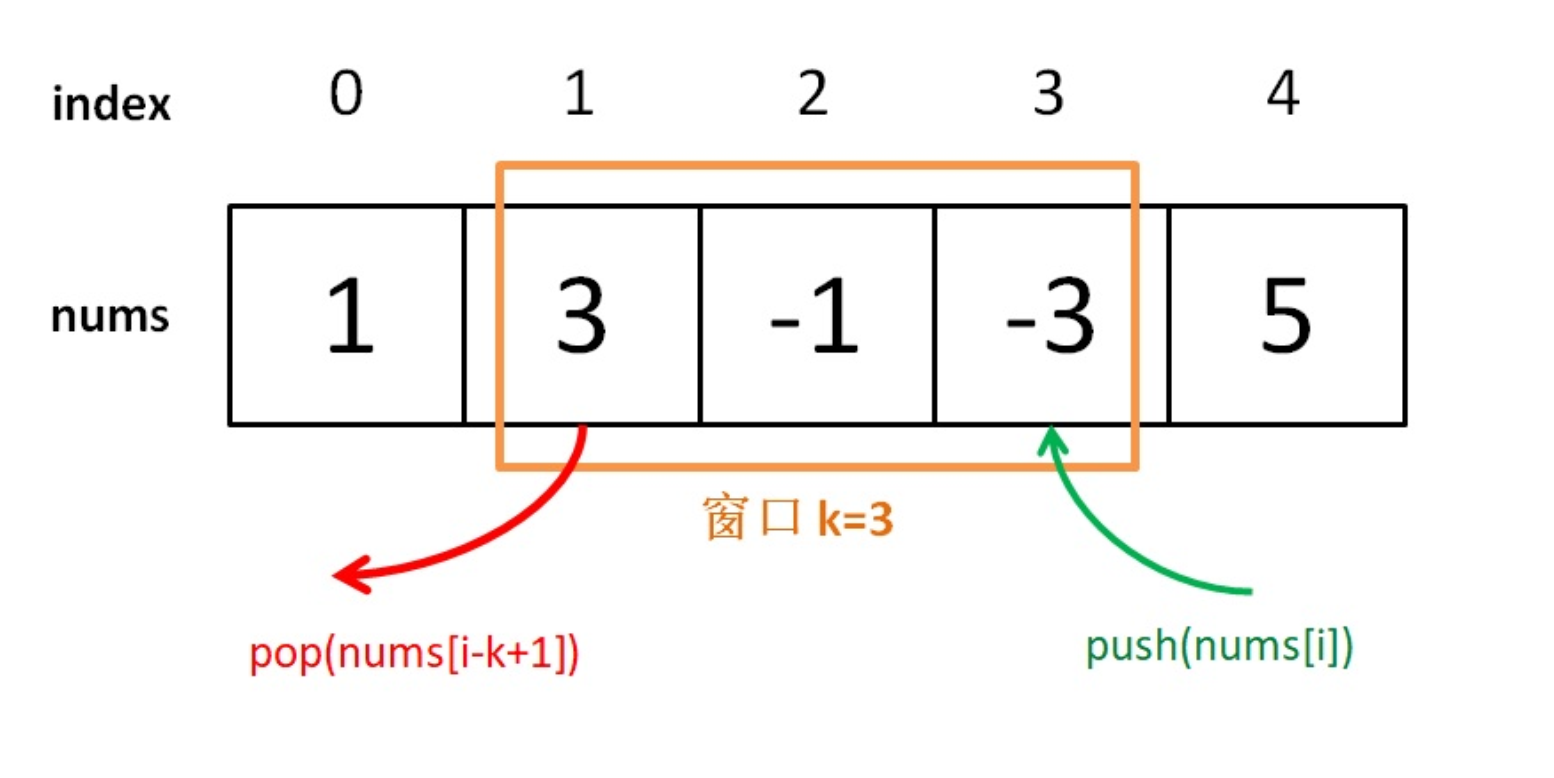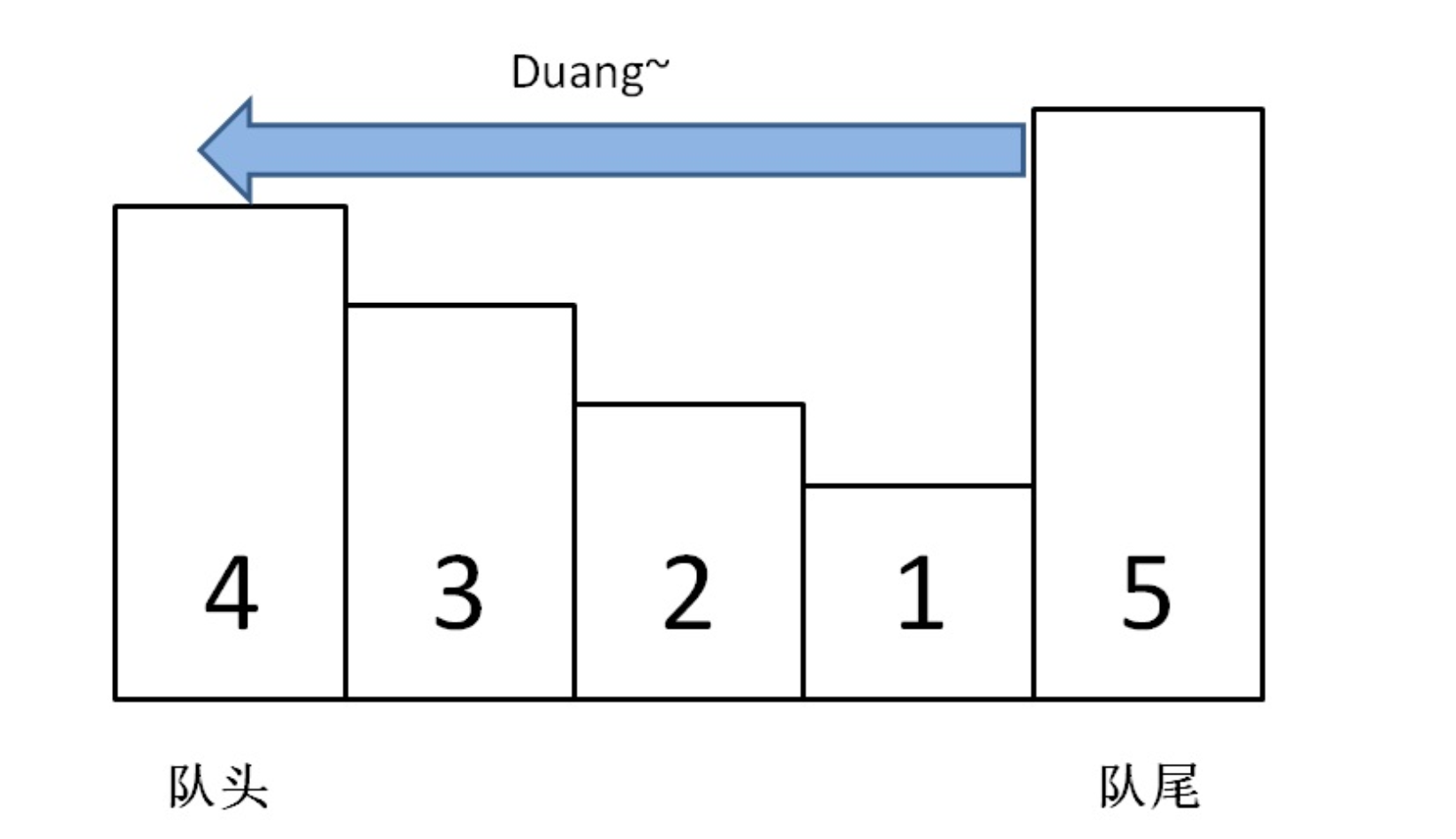• 累计撰写 270 篇文章
• 累计创建 6 个标签
• 累计收到 1 条评论

### 目 录CONTENT# 单调队列之滑动窗口.md2021-08-22 / 0 评论 / 0 点赞 / 6 阅读 / 2,560 字 / 正在检测是否收录...

## 单调队列之滑动窗口

输入: nums = [1,3,-1,-3,5,3,6,7], 和 k = 3

滑动窗口的位置                最大值
---------------               -----
[1  3  -1] -3  5  3  6  7       3
1 [3  -1  -3] 5  3  6  7       3
1  3 [-1  -3  5] 3  6  7       5
1  3  -1 [-3  5  3] 6  7       5
1  3  -1  -3 [5  3  6] 7       6
1  3  -1  -3  5 [3  6  7]      7


⼀、 搭建解题框架

⼀个「单调队列」 的操作也不多：

class MonotonicQueue {
// 在队尾添加元素 n
void push(int n);

// 返回当前队列中的最⼤值
int max();

// 队头元素如果是 n， 删除它
void pop(int n);
}

class Solution {
public:
vector<int> maxSlidingWindow(vector<int>& nums, int k) {
MonotonicQueue window;
vector<int> res;
for (int i = 0; i < nums.size(); i++) {
if (i < k - 1) { //先把窗⼝的前 k - 1 填满
window.push(nums[i]);
}
else
{ // 窗⼝开始向前滑动
window.push(nums[i]);
res.push_back(window.max());
window.pop(nums[i - k + 1]);
// nums[i - k + 1] 就是窗⼝最后的元素
}
}
return res;
}
};⼆、 实现单调队列数据结构

⾸先我们要认识另⼀种数据结构： deque， 即双端队列。 很简单：

class deque {
// 在队头插⼊元素 n
void push_front(int n);

// 在队尾插⼊元素 n
void push_back(int n);

// 在队头删除元素
void pop_front();

// 在队尾删除元素
void pop_back();

// 返回队头元素
int front();

// 返回队尾元素
int back();
};


单调队列」 的核⼼思路和「单调栈」 类似。 单调队列的 push ⽅法依然在队尾添加元素， 但是要把前⾯⽐新元素⼩的元素都删掉

class MonotonicQueue {

private:
deque<int> data;
public:
void push(int n)
{
while (!data.empty() && data.back() < n)
data.pop_back();
data.push_back(n);
}
};int max() {
return data.front();
}


pop() API 在队头删除元素 n， 也很好写：

void pop(int n) {
if (!data.empty() && data.front() == n)
data.pop_front();
}


⾄此， 单调队列设计完毕， 看下完整的解题代码：

class MonotonicQueue
{
private:
deque<int> data;
public:
void push(int n)
{
while (!data.empty() && data.back() < n)
data.pop_back();
data.push_back(n);
}
int max()
{
return data.front();
}

void pop(int n)
{
if (!data.empty() && data.front() == n)
data.pop_front();
}
};

vector<int> maxSlidingWindow(vector<int>& nums, int k)
{
MonotonicQueue window;
vector<int> res;

for (int i = 0; i < nums.size(); i++)
{
if (i < k - 1)
{ //先填满窗⼝的前 k - 1
window.push(nums[i]);
}
else
{ // 窗⼝向前滑动
window.push(nums[i]);
res.push_back(window.max());
window.pop(nums[i - k + 1]);
}
}
return res;
}


0